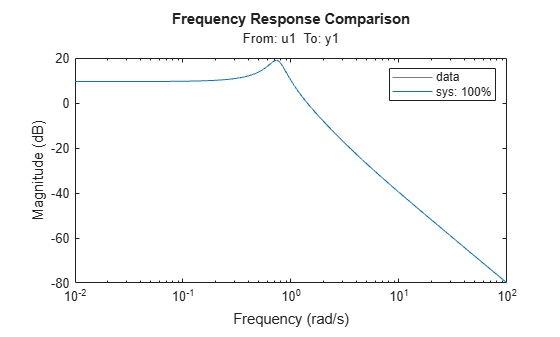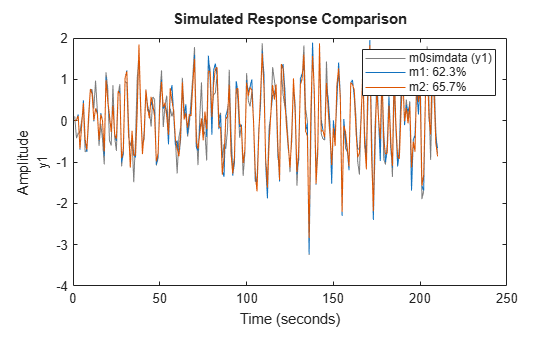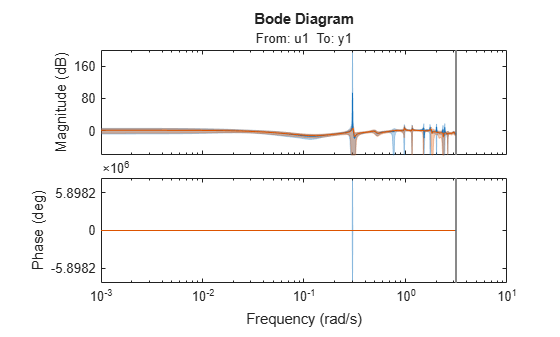Documentation

# oe

Estimate Output-Error polynomial model using time or frequency domain data

## Syntax

```sys = oe(data,[nb nf nk]) sys = oe(data,[nb nf nk],Name,Value) sys = oe(data,init_sys) sys = oe(data,___,opt) ```

## Description

```sys = oe(data,[nb nf nk])``` estimates an Output-Error model, `sys`, represented by:

`$y\left(t\right)=\frac{B\left(q\right)}{F\left(q\right)}u\left(t-nk\right)+e\left(t\right)$`

y(t) is the output, u(t) is the input, and e(t) is the error.

`sys` is estimated for the time- or frequency-domain, measured input-output data, `data`. The orders, ```[nb nf nk]```, parameterize the estimated polynomial.

```sys = oe(data,[nb nf nk],Name,Value)``` specifies model structure attributes using additional options specified by one or more `Name,Value` pair arguments.

`sys = oe(data,init_sys)` uses the linear system `init_sys` to configure the initial parameterization of `sys`.

`sys = oe(data,___,opt)` estimates a polynomial model using the option set, opt, to specify estimation behavior.

## Input Arguments

 `data` Estimation data. For time domain estimation, `data` is an `iddata` object containing the input and output signal values. For frequency domain estimation, `data` can be one of the following: Recorded frequency response data (`frd` or `idfrd`)`iddata` object with its properties specified as follows:`InputData` — Fourier transform of the input signal`OutputData` — Fourier transform of the output signal`Domain` — `'Frequency'` For multi-experiment data, the sample times and inter-sample behavior of all the experiments must match. `[nb nf nk]` Output error model orders. For a system represented by: `$y\left(t\right)=\frac{B\left(q\right)}{F\left(q\right)}u\left(t-nk\right)+e\left(t\right)$` where y(t) is the output, u(t) is the input and e(t) is the error. `nb` — Order of the B polynomial + 1. `nb` is an Ny-by-Nu matrix. Ny is the number of outputs and Nu is the number of inputs.`nf` — Order of the F polynomial. `nf` is an Ny-by-Nu matrix. Ny is the number of outputs and Nu is the number of inputs.`nk` — Input delay, expressed as the number of samples. `nk` is an Ny-by-Nu matrix. Ny is the number of outputs and Nu is the number of inputs. The delay appears as leading zeros of the B polynomial. For estimation using continuous-time data, only specify ```[nb nf]``` and omit `nk`. `init_sys` Linear system that configures the initial parameterization of `sys`. You obtain `init_sys` by either performing an estimation using measured data or by direct construction. If `init_sys` is an `idpoly` model of Output-Error structure, `oe` uses the parameter values of `init_sys` as the initial guess for estimating `sys`. Use the `Structure` property of `init_sys` to configure initial guesses and constraints for B(q) and F(q). For example: To specify an initial guess for the F(q) term of `init_sys`, set `init_sys.Structure.F.Value` as the initial guess. To specify constraints for the B(q) term of `init_sys`:Set `init_sys.Structure.B.Minimum` to the minimum B(q) coefficient valuesSet `init_sys.Structure.B.Maximum` to the maximum B(q) coefficient valuesSet `init_sys.Structure.B.Free` to indicate which B(q) coefficients are free for estimation If `init_sys` is not a polynomial model of Output-Error structure, the software first converts `init_sys` to an Output-Error structure model. `oe` uses the parameters of the resulting model as the initial guess for estimating `sys`. If `opt` is not specified, and `init_sys` was created by estimation, then the estimation options from `init_sys.Report.OptionsUsed` are used. `opt` Estimation options. `opt` is an option set, created using `oeOptions`, that specifies estimation options including: Estimation objectiveHandling of initial conditionsNumerical search method and the associated options

### Name-Value Pair Arguments

Specify optional comma-separated pairs of `Name,Value` arguments. `Name` is the argument name and `Value` is the corresponding value. `Name` must appear inside quotes. You can specify several name and value pair arguments in any order as `Name1,Value1,...,NameN,ValueN`.

 `'InputDelay'` Input delay for each input channel, specified as a scalar value or numeric vector. For continuous-time systems, specify input delays in the time unit stored in the `TimeUnit` property. For discrete-time systems, specify input delays in integer multiples of the sample time `Ts`. For example, ```InputDelay = 3``` means a delay of three sample times. For a system with `Nu` inputs, set `InputDelay` to an `Nu`-by-1 vector. Each entry of this vector is a numerical value that represents the input delay for the corresponding input channel. You can also set `InputDelay` to a scalar value to apply the same delay to all channels. Default: 0 `'IODelay'` Transport delays. `IODelay` is a numeric array specifying a separate transport delay for each input/output pair. For continuous-time systems, specify transport delays in the time unit stored in the `TimeUnit` property. For discrete-time systems, specify transport delays as integers denoting delay of a multiple of the sample time `Ts`. You can specify `IODelay` as an alternative to the `nk` value. Doing so simplifies the model structure by reducing the number of leading zeros the B polynomial. In particular, you can represent `max(nk-1,0)` leading zeros as input/output delays using `IODelay` instead. For a MIMO system with `Ny` outputs and `Nu` inputs, set `IODelay` to a `Ny`-by-`Nu` array. Each entry of this array is a numerical value that represents the transport delay for the corresponding input/output pair. You can also set `IODelay` to a scalar value to apply the same delay to all input/output pairs. Default: `0` for all input/output pairs

## Output Arguments

`sys`

Output-Error polynomial model that fits the estimation data, returned as a `idpoly` model object. This model is created using the specified model orders, delays, and estimation options.

Information about the estimation results and options used is stored in the `Report` property of the model. `Report` has the following fields:

Report FieldDescription
`Status`

Summary of the model status, which indicates whether the model was created by construction or obtained by estimation.

`Method`

Estimation command used.

`InitialCondition`

Handling of initial conditions during model estimation, returned as one of the following values:

• `'zero'` — The initial conditions were set to zero.

• `'estimate'` — The initial conditions were treated as independent estimation parameters.

• `'backcast'` — The initial conditions were estimated using the best least squares fit.

This field is especially useful to view how the initial conditions were handled when the `InitialCondition` option in the estimation option set is `'auto'`.

`Fit`

Quantitative assessment of the estimation, returned as a structure. See Loss Function and Model Quality Metrics for more information on these quality metrics. The structure has the following fields:

FieldDescription
`FitPercent`

Normalized root mean squared error (NRMSE) measure of how well the response of the model fits the estimation data, expressed as a percentage.

`LossFcn`

Value of the loss function when the estimation completes.

`MSE`

Mean squared error (MSE) measure of how well the response of the model fits the estimation data.

`FPE`

Final prediction error for the model.

`AIC`

Raw Akaike Information Criteria (AIC) measure of model quality.

`AICc`

Small sample-size corrected AIC.

`nAIC`

Normalized AIC.

`BIC`

Bayesian Information Criteria (BIC).

`Parameters`

Estimated values of model parameters.

`OptionsUsed`

Option set used for estimation. If no custom options were configured, this is a set of default options. See `oeOptions` for more information.

`RandState`

State of the random number stream at the start of estimation. Empty, `[]`, if randomization was not used during estimation. For more information, see `rng` in the MATLAB® documentation.

`DataUsed`

Attributes of the data used for estimation, returned as a structure with the following fields:

FieldDescription
`Name`

Name of the data set.

`Type`

Data type.

`Length`

Number of data samples.

`Ts`

Sample time.

`InterSample`

Input intersample behavior, returned as one of the following values:

• `'zoh'` — Zero-order hold maintains a piecewise-constant input signal between samples.

• `'foh'` — First-order hold maintains a piecewise-linear input signal between samples.

• `'bl'` — Band-limited behavior specifies that the continuous-time input signal has zero power above the Nyquist frequency.

`InputOffset`

Offset removed from time-domain input data during estimation. For nonlinear models, it is `[]`.

`OutputOffset`

Offset removed from time-domain output data during estimation. For nonlinear models, it is `[]`.

`Termination`

Termination conditions for the iterative search used for prediction error minimization. Structure with the following fields:

FieldDescription
`WhyStop`

Reason for terminating the numerical search.

`Iterations`

Number of search iterations performed by the estimation algorithm.

`FirstOrderOptimality`

$\infty$-norm of the gradient search vector when the search algorithm terminates.

`FcnCount`

Number of times the objective function was called.

`UpdateNorm`

Norm of the gradient search vector in the last iteration. Omitted when the search method is `'lsqnonlin'` or `'fmincon'`.

`LastImprovement`

Criterion improvement in the last iteration, expressed as a percentage. Omitted when the search method is `'lsqnonlin'` or `'fmincon'`.

`Algorithm`

Algorithm used by `'lsqnonlin'` or `'fmincon'` search method. Omitted when other search methods are used.

For estimation methods that do not require numerical search optimization, the `Termination` field is omitted.

For more information on using `Report`, see Estimation Report.

## Examples

collapse all

Obtain the estimation data.

```filename = fullfile(matlabroot,'examples','ident','oe_data1.mat'); load(filename);```

`data`, an `idfrd` object, contains the continuous-time frequency response for the following model:

`$G\left(s\right)=\frac{s+3}{{s}^{3}+2{s}^{2}+s+1}$`

Estimate the model.

```nb = 2; nk = 3; sys = oe(data,[nb nk]);```

Evaluate the goodness of the fit.

`compare(data,sys);`Estimate a high-order OE model from data collected by simulating a high-order system. Determine the regularization constants by trial and error and use the values for model estimation.

`load regularizationExampleData.mat m0simdata`

Estimate an unregularized OE model of order 30.

`m1 = oe(m0simdata,[30 30 1]);`

Obtain a regularized OE model by determining Lambda value using trial and error.

```opt = oeOptions; opt.Regularization.Lambda = 1; m2 = oe(m0simdata,[30 30 1],opt);```

Compare the model outputs with the estimation data.

```opt = compareOptions('InitialCondition','z'); compare(m0simdata,m1,m2,opt);```The regularized model, `m2`, produces a better fit than the unregularized model, `m1`.

Compare the variance in the model responses.

```h = bodeplot(m1,m2); opt = getoptions(h); opt.PhaseMatching = 'on'; opt.ConfidenceRegionNumberSD = 3; opt.PhaseMatching = 'on'; setoptions(h,opt); showConfidence(h);```The variance of the regularized model `m2` is reduced compared to the unregularized model `m1`.

Obtain the estimation data.

```filename = fullfile(matlabroot,'examples','ident','oe_data2.mat'); load(filename,'data','Ts');```

`data`, an `iddata` object, contains the discrete-time frequency response for the following model:

`$G\left(s\right)=\frac{1000}{s+500}$`

The sample time for `data`, `Ts`, is 0.001 seconds.

Treat `data` as continuous-time data. When you plot `data`, the input/output signals are band-limited, which allows you to treat `data` as continuous-time data. You can now obtain a continuous-time model.

`data.Ts = 0;`

Specify the estimation options.

`opt = oeOptions('WeightingFilter',[0 0.5*pi/Ts]);`

This prefilter choice directs the software to ignore the response values for frequencies higher than `0.5*pi/Ts` rad/s.

Estimate the model.

```nb = 1; nf = 3; sys = oe(data,[nb nf],opt);```

collapse all

### Output-Error (OE) Model

The general Output-Error model structure is:

`$y\left(t\right)=\frac{B\left(q\right)}{F\left(q\right)}u\left(t-{n}_{k}\right)+e\left(t\right)$`

The orders of the Output-Error model are:

### Continuous-Time, Output-Error Model

If `data` is continuous-time frequency-domain data, `oe` estimates a continuous-time model with transfer function:

`$G\left(s\right)=\frac{B\left(s\right)}{F\left(s\right)}=\frac{{b}_{nb}{s}^{\left(nb-1\right)}+{b}_{nb-1}{s}^{\left(nb-2\right)}+...+{b}_{1}}{{s}^{nf}+{f}_{nf}{s}^{\left(nf-1\right)}+...+{f}_{1}}$`

The orders of the numerator and denominator are `nb` and `nf`, similar to the discrete-time case. However, the delay `nk` has no meaning and you should omit it when specifying model orders for estimation. Use `model = oe(data,[nb nf])`. Use the `IODelay` model property to specify any input-output delays. For example, use ```model = oe(data,[nb nf], 'IODelay',iod)``` instead.

## Tips

• To estimate a continuous-time model when `data` represents continuous-time frequency response data, omit `nk`.

For example, use `sys = oe(data,[nb nf])`.

## Algorithms

The estimation algorithm minimizes prediction errors.

## Alternatives

Output-Error models are a special configuration of polynomial models, having only two active polynomials - B and F. For such models, it may be more convenient to use a transfer function (`idtf`) model and its estimation command, `tfest`.

Also, `tfest` is the recommended command for estimating continuous-time models.

## Extended Capabilities